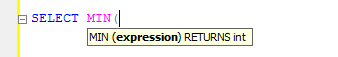﻿ How to use MIN function inside Where Clause in SQL Server

# How to use MIN function inside Where Clause in SQL Server

The aggregate MIN() function in SQL Server is used to find the minimum or the smallest value of a column. It is a very commonly used built-in function in SQL Server. Now recently I came across a situation where I had to use the MIN function in a WHERE clause. I’ll show you how this is done.

## The MIN() Function

### Syntax

SELECT MIN(expression) from table

The MIN() function takes a parameter in the form of an expression (or a column name). It returns an int.Normally, we’ll use the MIN() inside a SELECT statement to fetch the minimum or the lowest value of a given column.

For example, I have a table named ProductDetails.

```ID  Brand       Price   Quantity
1   Natrag      20      110
2   Mono        15      200
3   Elxin       17.5    121
4   Parcc       275     10
5   Smart       22      87
6   Doms        40      11
7   X-Presso    175     65```

Now suppose, if I want to get the lowest value of the Quantity column, I’ll use the function like this.

SELECT MIN(Quantity) MinQty FROM ProductDetails

The result would be
10

You can use the MIN() function is a variety of ways. One of the ways, is using it inside a WHERE clause.

You must also read this: How to use the LEFT() and RIGHT() functions in SQL Server and why use these functions?

## Using MIN() inside WHERE clause

I am using the same table above for this example. I want to find the Max value (Quantity) between the minimum and maximum values. It’s kind of a range where I know the maximum value in the range, but I want the fetch the minimum value dynamically. Here’s how I’ll do it.
```SELECT MAX(Quantity) MaxQty FROM ProductDetails
WHERE Quantity
BETWEEN (SELECT MIN(Quantity) FROM ProductDetails) and 150```

The result will be
121

Simple, isn't it. You can use the SQL Server MAX() function similarly to get a result.